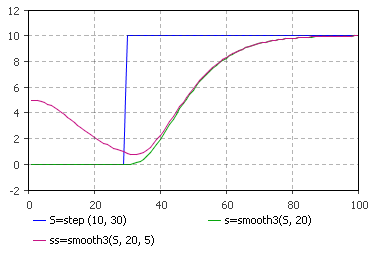AnyLogic

# smooth3

smooth3 is the system dynamics function that returns a third order exponential smooth of the input. It is frequently used to model information delays.

The plot below illustrates how the function works:smooth3 can be called in formulas of system dynamics variables and has two notations:

• smooth3(input, delayTime, initialValue)

input can be a flow variable, or a numeric expression of any complexity.

delayTime can be either a constant or a numeric expression (e.g. a function call, or a numeric parameter). It should return a positive number: smooth3 function with zero or negative delay time returns not a number.

• smooth3(input, delayTime)

The simplified notation of the function. Is used when the initial value is zero.

#### Units

input — unit

delayTime — time

initialValue — unit

trend() — unit

The output units are the same as the input ones.